# AP Chemistry : Solutions

## Example Questions

← Previous 1 3 4 5 6 7 8 9 10

### Example Question #1 : Colligative Properties

Which of the following solutions has the highest boiling point?

Assume that all solutes in solution are nonvolatile.

1m sodium chloride

2m glucose

1m magnesium chloride

1m glucose

1m magnesium chloride

Explanation:

The equation for boiling point elevation is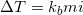.

Since all of the solutions are aqueous, we do not need to consider the boiling point elevation constant (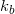) when comparing the solutions. The two factors we need to consider are molality () and the van't Hoff factor () of the solute.

Glucose will not ionize in solution, sodium chloride will make two ions in solution, and magnesium chloride will make three ions in solution.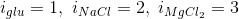When multiplying the molality by the van't Hoff factor, we can determine that the magnesium chloride solution will elevate the boiling point by the highest number.

### Example Question #2 : Colligative Properties

What is the boiling point of a solution created when four moles of glucose are dissolved in two kilograms of water?

Assume that glucose is a nonvolatile solute.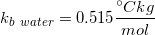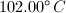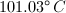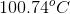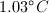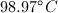Explanation:

Since the glucose is nonvolatile, we can use the boiling point elevation equation to solve for the new boiling point.Since glucose does not ionize in water, the van't Hoff factor is simply 1 for this problem. The molality can be found by the moles of solute per kilogram of solvent.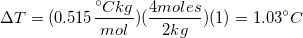This means that the boiling point for the water will be elevated by 1.03oC with the addition of the glucose. Since pure water has a boiling point of 100oC, the boiling point for this solution is 101.03oC.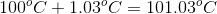### Example Question #3 : Colligative Properties

Which equation accurately describes what happens to the boiling point when a solute is added to a liquid? (K = constant, M = molarity, m = molality)

delta T = Mm/K
delta T = K/m
delta T = K/M
delta T = K*M
delta T = K*m
Correct answer: delta T = K*m
Explanation:

The correct answer choice is the equation for boiling point elevation when solute is added to a solvent.

### Example Question #4 : Colligative Properties

50g of an unknown compound are added to three kilograms of water. The compound is nonvolatile, and has a van't Hoff factor of 2. It is determined that the solution has a freezing point of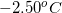.

What is the molar mass of the unknown compound?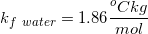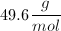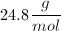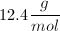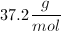Explanation:

In order to solve for the molar mass of the unknown compound, we need to use the freezing point depression equation.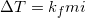Since molality is equal to the moles of solute divided by the kilograms of solvent, we can substitute the moles of solute with the mass of the solute divided by the molar mass of the solute.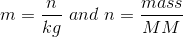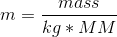This allows us to solve for the molar mass of the compound using a substituted equation.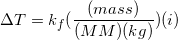We can use the values given in the question to solve for the molar mass.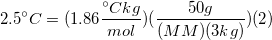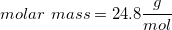### Example Question #5 : Colligative Properties

What is the freezing point of a 2M solution of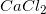in water?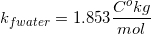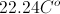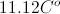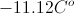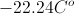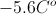Explanation: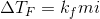First, we need to calculate the molality because that is what we use in our equation for freezing point depression. We can get that from the molarity without knowing exactly how many liters or grams we have. We just have to know what we have one mole per liter. The weight of water is one kilogram per liter, so this allows us to make this conversion.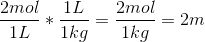The molality is 2m. The van't Hoff factor is 3, as we get one calcium ion and two chloride ions per molecule during dissociation.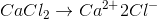We can now plug the values into the equation for freezing point depression.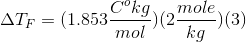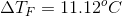This gives us our depression of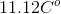.  The normal freezing point of pure water is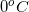, which means our new freezing point is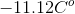.

### Example Question #6 : Colligative Properties

What is the new freezing point of 3L of aqueous solution that contains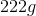of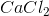and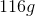of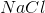?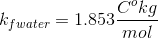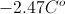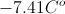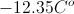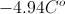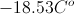Explanation: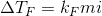The important thing to remember for this question is that it doesn't matter what the solutes are in freezing point depression, just how many ions are created during dissociation. First, we need to convert our solute amounts to moles.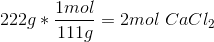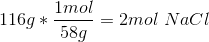The molality of the solution is the moles of solute per kilogram of solvent. Since water has a density of one kilogram per liter, we can simply divide the total moles by the liters of solution. The molality of the solution is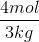.

We can treat this solution as 3L of water containing 4mol of a solute that dissolves into a total of 5 ions. It does not matter which compound the ions come from, only that they end up in solution in the correct proportion. 2mol of calcium chloride will contribute 3 ions per molecule and 2mol of sodium chloride will contribute 2 ions per molecule, for a total of 5 ions per mole of solution.

Using these conclusions, we can solve the freezing point depression.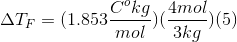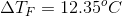Our depression is then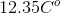. Since the freezing point of pure water is, our new temperature for freezing point is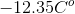.

### Example Question #7 : Colligative Properties

A solution ofof water and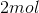of glucose has a freezing point depression of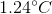. Which of the following changes would NOT triple the original change in the melting point?

Placeof glucose in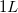of water

Replaceof glucose with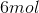ofTriple the glucose amount

Replace the glucose withof magnesium chloride

Replaceof glucose withofExplanation:

For reference, the freeing point depression equation is: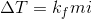Since we want to triple the melting point change, we need to manipulate the freezing point depression equation so that the value ofis three times as large.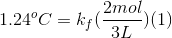Replacing glucose with magnesium chloride will make the van't Hoff factor three, so the melting point change will triple.

Reducing the amount of water by a factor of three will also triple the melting point change, as will tripling the glucose amount.

Six moles of sodium chloride will triple the solute concentration and make the van't Hoff factor two. This multiplies the melting point change by six.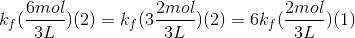### Example Question #8 : Colligative Properties

It is snowing outside and you decide to throw salt onto the driveway. What is the purpose of throwing salt onto the driveway?

The salt will displace the water molecules, releasing them from the concrete

The salt will dissolve into the water from the snow, causing the freezing point to decrease

The salt and snow will repel each other, removing the water molecules form the area

The only purpose of the salt is to create better traction, so that walking on the driveway is safer

The salt ions will dissociate and create a hydrophobic environment, causing the snow not to stick on the driveway

The salt will dissolve into the water from the snow, causing the freezing point to decrease

Explanation:

When a solid is dissolved into a liquid, in our case water (snow), the freezing point will decrease. This phenomenon is called freezing point depression. In order to freeze, the molecules of the liquid must organize into a relatively static lattice. This highly organized structure is disrupted by the presence of extra molecules and ions that are dissolved in the liquid, making it harder to transition to a solid.

With enough salt in the snow, the freezing point will depress below the current temperature, leading the snow to melt and be in the liquid phase. The salt ions will infiltrate the ice/snow lattice, breaking apart the solid and cause the water to remain liquid.

### Example Question #9 : Colligative Properties

You are attempting to distill the water from a sample of seawater. What can you do to facilitate the process?

Filter the water to remove some solute

Add more salt into the water

Increase the pressure

There is no way to affect the boiling point of the sample

Move to a lower elevation

Filter the water to remove some solute

Explanation:

Dissolving solutes into a solvent will influence the movement of the molecules in solution. In a pure solvent, the molecules are essentially in uniform motion. When an impurity is added, such as salt in seawater, the foreign particles begin to interact with the solvent. Different masses, velocities, and attractive forces alter the patterns of the liquid molecules and cause an overall decrease in their energy. Transitioning to a gaseous state from a liquid state requires a reduction of intermolecular forces, while adding solute increases these forces in the solution. Adding solute thus inhibits the transition from liquid to gas, resulting in boiling point elevation.

Filtering the water to remove some solute could help to distill the water by removing some of the boiling point elevation. Moving to the lower elevation or increasing the pressure will increasing the boiling point.

### Example Question #10 : Colligative Properties

The vapor pressure of ethanol at room temperature is 45mmHg. A nonvolatile solute is added to a vial of ethanol, resulting in a solution with a vapor pressure of 34mmHg. What is the molar fraction of the nonvolatile solute in the solution?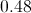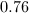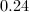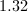Explanation:

A nonvolatile solute will not contribute to the vapor pressure of a solution, and will only act to decrease the vapor pressure of the pure solvent. The molar fraction of the solvent in the solution can be determined using Raoult's law.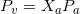The solution's vapor pressure is equal to the vapor pressure of the pure solvent multiplied by the molar fraction of solvent in solution.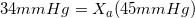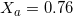This means that the molar fraction of solvent in the solution is 0.76. As a result, we conclude that the molar fraction of solute in the solution is 0.24, since the sum of the mole fractions must equal 1.

← Previous 1 3 4 5 6 7 8 9 10